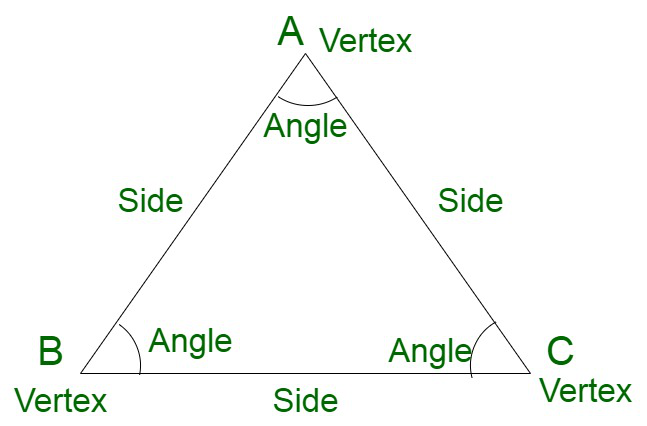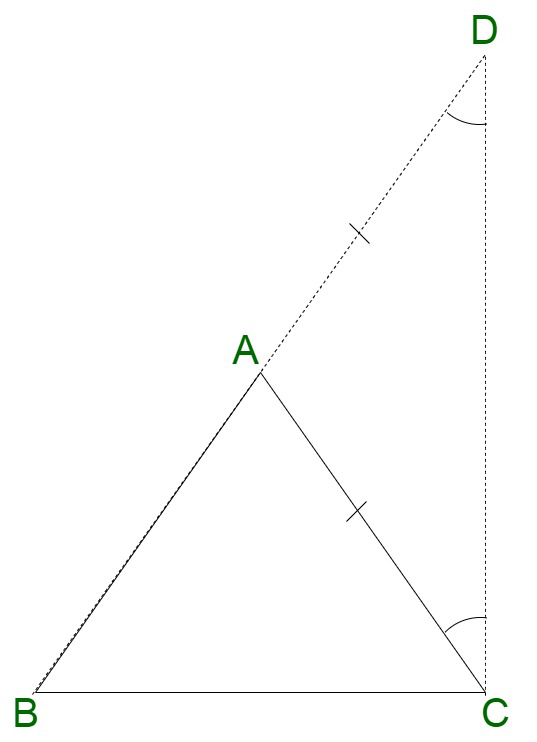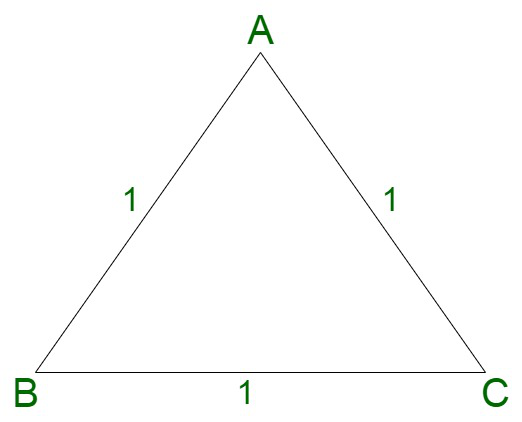Open in App
Not now

# Prove that the sum of any two sides of a triangle be greater than the third side

• Last Updated : 28 Nov, 2021

Geometry is the branch of mathematics that deals with the study of different types of shapes and figures and sizes. The branch of geometry deals with different angles, transformations, and similarities in the figures seen.

Triangle

A triangle is a closed two-dimensional shape associated with three angles, three sides, and three vertices. A triangle associated with three vertices says A, B, and C is represented as △ABC. It can also be termed as a three-sided polygon or trigon. Some of the common examples of triangles are signboards and sandwiches.### Prove that the sum of any two sides of a triangle be greater than the third side

To prove: The sum of two sides of a triangle is greater than the third side, BA + AC > BC

Assume: Let us assume ABC to be a triangle.Proof:

Extend the line segment BA to D,

⇒ ∠ ADC = ∠ ACD

Observing by the diagram, we obtain,

∠ DCB > ∠ ACD

⇒ ∠ DCB > ∠ ADC

⇒ BD > AB (Since the sides opposite to the larger angle is larger and the sides opposite to smaller angle is smaller)

⇒ BA + AD > BC

⇒ BA + AC > BC.

Hence proved.

Note: Similarly it can be also proved that, BA + BC > AC or AC + BC > BA

Hence, The sum of two sides of a triangle is greater than the third side.

### Sample Questions

Question 1. Prove that the above property holds for the lowest positive integral value.

Solution:

Let us assume ABC to be a triangle.

Each of the sides is 1 unit.

Now,

It is an equilateral triangle where all the sides are 1 each.

Taking sum of two sides,

AB + BC ,

1 + 1 > BC

1+1 > 1

2 > 1Question 2. Illustrate this property for a right-angled triangle

Solution:

Let us assume the sides of the right angles triangle to be 5,12 and 13.

Now,

Taking the smaller two sides, we obtain,

5 + 12 > 13

17 > 13

Hence, the property holds.

Question 3. Does this property hold for isosceles triangles?

Solution:

Let us assume a triangle with sides 2x, 2x, and x.

Now,

Taking the sum of equal two sides, we obtain,

2x + 2x = 4x

which is greater than the third side, equivalent to x.

My Personal Notes arrow_drop_up